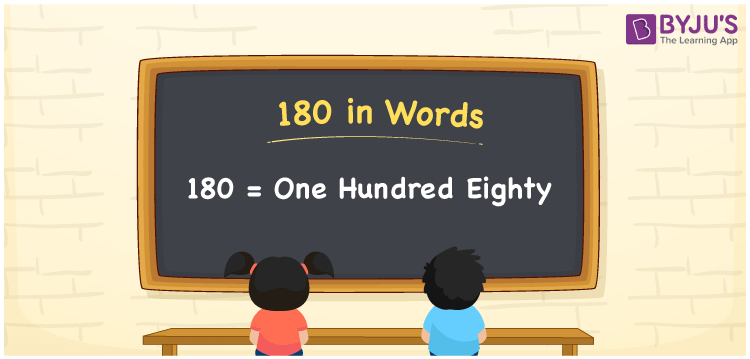# 180 in Words

The word one hundred eighty is used to represent the number 180. Using the place value of a number, the number 180 can be easily converted into words. As a result, a place value table is required to write the number names. For example, if you spent Rs. 180 for buying a book, you can write “I spent Rs. One hundred eighty for buying a book”. This article will teach you how to spell 180 in English as well as how to describe the number 180 in words.

 180 in Words: One Hundred Eighty. One Hundred Eighty in Numerical Form: 180.

## 180 in English Words## How to Write 180 in Words?

The table below shows the place value for the number 180.

 Hundreds Tens Ones 1 8 0

The following is the expanded version of the number 180:

= 1 × Hundred + 8 × Ten + 0 × One

= 1 × 100 + 8 × 10

= 100 + 80

= 180

= One hundred eighty

Hence, 180 in words is One hundred eighty.

180 in words – One hundred eighty

Is 180 an odd number? – No

Is 180 an even number? – Yes

Is 180 a perfect square number? – No

Is 180 a perfect cube number? – No

Is 180 a prime number? – No

Is 180 a composite number? – Yes

## Frequently Asked Questions on 180 in Words

Q1

### Write 180 in words.

180 in words is one hundred eighty.

Q2

### Simplify 100 + 80, and express in words.

Simplifying 100 + 80, we get 180. Hence, 180 in words is one hundred eighty.

Q3

### How to write one hundred eighty in numbers?

One hundred eighty in numbers is 180.